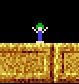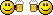###Author Topic: Point inside polygon  (Read 4200 times)

####doimus

• Dr. Type
••• Posts: 284##### Point inside polygon
« on: 2011-Oct-09 »
Here's some code that finds whether a particular point is inside a polygon.
Actual routine is not mine, I've found the C code online (http://www.visibone.com/inpoly/inpoly.c) and translated it into GLB.

It's great for implementing various regions, zones, collision or whatever else into your programs.
Code is pure math, it has nothing to do with graphical polygons (no acceleration) but it supports all kinds of mad polygonal shapes.

Code: GLBasic [Select]
SYSTEMPOINTER TRUE

//create polygon
LOCAL poly[]
DIM poly[RND(3)+3]

FOR i = 0 TO BOUNDS(poly[], 0) - 1
poly[i] = RND(640)
poly[i] = RND(480)
NEXT

// main loop
WHILE TRUE

LOCAL mx,my,mba,mbb
MOUSESTATE mx, my, mba, mbb

drawPoly(poly[])

IF inPoly(poly[], mx, my)
PRINT "Inside!",0,0
ENDIF

SHOWSCREEN

WEND

FUNCTION inPoly: poly[], tx, ty

LOCAL newx, newy, oldx, oldy
LOCAL x1, y1, x2, y2
LOCAL inside

LOCAL npoints = BOUNDS(poly[], 0)

IF npoints < 3 THEN RETURN 0

oldx = poly[npoints - 1]
oldy = poly[npoints - 1]

FOR i = 0 TO npoints - 1

newx = poly[i]
newy = poly[i]

IF newx > oldx

x1 = oldx
x2 = newx
y1 = oldy
y2 = newy

ELSE

x1 = newx
x2 = oldx
y1 = newy
y2 = oldy

ENDIF

IF ((newx < tx) = (tx <= oldx) AND (ty-y1)*(x2-x1) < (y2-y1)*(tx-x1))

inside = NOT inside

ENDIF

oldx = newx
oldy = newy

NEXT

RETURN inside

ENDFUNCTION

FUNCTION drawPoly: poly[]

LOCAL npoints = BOUNDS(poly[], 0)

FOR i = 0 TO npoints-2
DRAWLINE poly[i],poly[i], poly[i+1],poly[i+1], RGB(200,200,200)
NEXT

DRAWLINE poly[-1],poly[-1], poly,poly, RGB(200,200,200)

ENDFUNCTION
« Last Edit: 2014-May-03 by Moru »

####AMateus

• Mr. Drawsprite
•• Posts: 61##### Re: Point inside polygon
« Reply #1 on: 2011-Oct-09 »
NiceThank you for sharing.

####matchy

• Prof. Inline
••• Posts: 1542##### Re: Point inside polygon
« Reply #2 on: 2011-Oct-10 »
Cool. Looks faster than the code I'd converted from http://www.vb-helper.com/howto_find_angles.html

Code: GLBasic [Select]

TYPE _vertex
pts[] // polygon
x
y
z
width
height
off_x
off_y
ENDTYPE

FUNCTION PointInPolygon: ply AS _vertex, point AS _vertex
LOCAL c, corners, total_angle
LOCAL ver AS _vertex

corners = BOUNDS(ply.pts[], 0) - 1
total_angle = GetAngleProduct(ply.pts[corners], ply.pts[corners], point.x, point.y, ply.pts, ply.pts)
FOR c = 0 TO corners - 1
ver.x = ply.pts[c] + ply.x
ver.y = ply.pts[c] + ply.y
ver.off_x = point.x
ver.off_y = point.y
ver.width = ply.pts[c + 1] + ply.x
ver.height = ply.pts[c + 1] + ply.y
total_angle = total_angle + GetAngleProduct(ver.x, ver.y, ver.off_x, ver.off_y, ver.width, ver.height)
NEXT
RETURN ABS(INTEGER(total_angle))
ENDFUNCTION

FUNCTION GetAngleProduct: Ax,Ay,Bx,By,Cx,Cy
LOCAL dot_product, cross_product

dot_product = DotProduct(Ax, Ay, Bx, By, Cx, Cy)
cross_product = CrossProductLength(Ax, Ay, Bx, By, Cx, Cy)
RETURN ATan2(cross_product, dot_product)
ENDFUNCTION

LOCAL angle
LOCAL iPI = 3.14159265358979323846

angle = iPI / 2
ELSE
ENDIF
angle  = iPI - angle
ENDIF
IF opp < 0
angle = -angle
ENDIF
RETURN angle
ENDFUNCTION

FUNCTION CrossProductLength: Ax,Ay,Bx,By,Cx,Cy
LOCAL BAx=Ax-Bx
LOCAL BAy=Ay-By
LOCAL BCx=Cx-Bx
LOCAL BCy=Cy-By
RETURN (BAx*BCy) - (BAy*BCx)
ENDFUNCTION

FUNCTION DotProduct: Ax,Ay,Bx,By,Cx,Cy
LOCAL BAx= Ax - Bx
LOCAL BAy= Ay - By
LOCAL BCx= Cx - Bx
LOCAL BCy= Cy - By
RETURN (BAx*BCx) + (BAy*BCy)
ENDFUNCTION

####Wampus

• Prof. Inline
••• Posts: 1004##### Re: Point inside polygon
« Reply #3 on: 2012-Apr-19 »
Cheers me dears. I was just about to write a routine to do this myself. Now I won't have to.####xbee

• Mc. Print
•• Posts: 10##### Re: Point inside polygon
« Reply #4 on: 2013-Nov-11 »
Perfect. Just what I was looking forMany thanks.

####mentalthink

• Prof. Inline
••• Posts: 3366
• Integrated Brain##### Re: Point inside polygon
« Reply #5 on: 2013-Nov-12 »
Thanks -domius very interesting the code, perhaps for void make a for over  a huge mesh can works fine?¿...
Thanks I think I use your code in my simple 3D Editor...